# Which of the following components of a linear programming model is the overall performance measure? Multiple...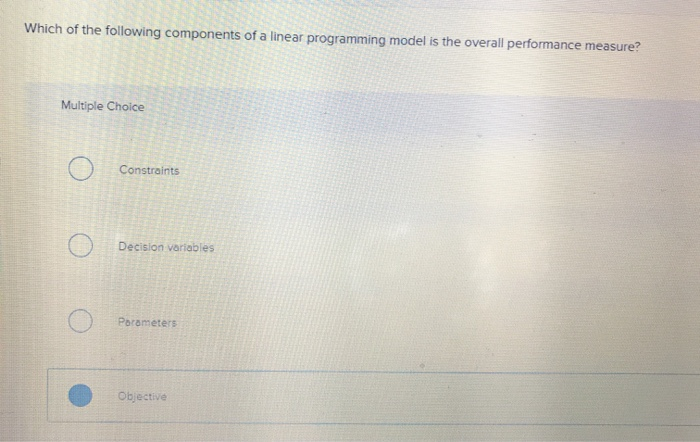Which of the following components of a linear programming model is the overall performance measure? Multiple Choice O Constraints Decision variables O Parameters Objective

Note : In the linear programming model, objective component represents the affect of decision variable upon the value and cost while determining the performance of the variables as constraints and objective functions effect changes that happens to one component as they are proportional to its magnitude.

#### Earn Coin

Coins can be redeemed for fabulous gifts.

Similar Homework Help Questions
• ### Solve the following model using linear programming (allow for continuous values and determine the values of...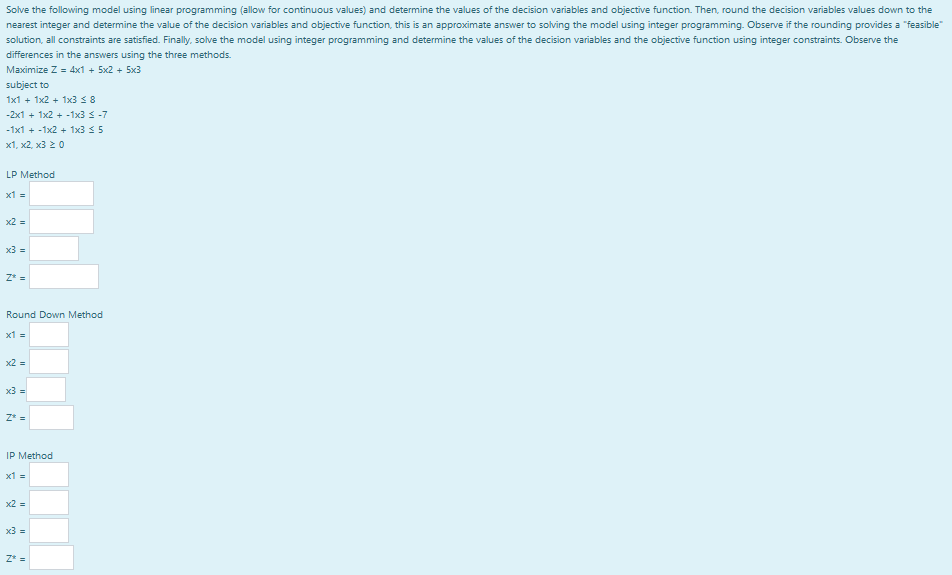Solve the following model using linear programming (allow for continuous values and determine the values of the decision variables and objective function. Then, round the decision variables values down to the nearest integer and determine the value of the decision variables and objective function, this is an approximate answer to solving the model using integer programming. Observe if the rounding provides a "feasible solution, all constraints are satisfied. Finally, solve the model using integer programming and determine the values of...

• ### Create a worksheet called "Transport a. Enter the following transportation model into excel following the linear...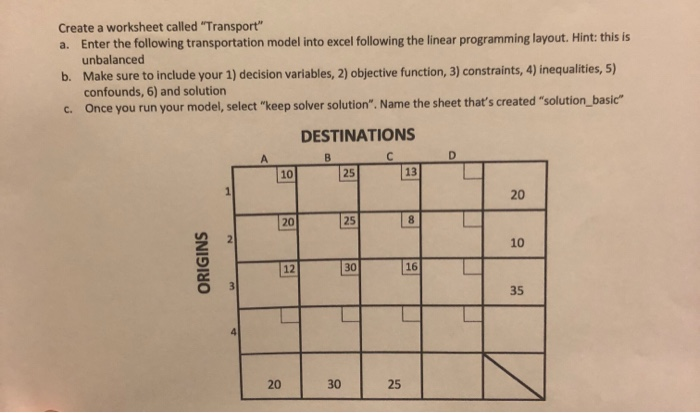Create a worksheet called "Transport a. Enter the following transportation model into excel following the linear programming layout. Hint: this is unbalanced Make sure to include your 1) decision variables, 2) objective function, 3) constraints, 4) inequalities, 5) confounds, 6) and solution b. c. Once you run your model, select "keep solver solution". Name the sheet that's created "solution_basic" DESTINATIONS 13 20 20 25 10 12 30 16 35 20 30 25

• ### Consider the following simple linear regression model: y=Po+P1x Po and B1 are Multiple Choice 41 the...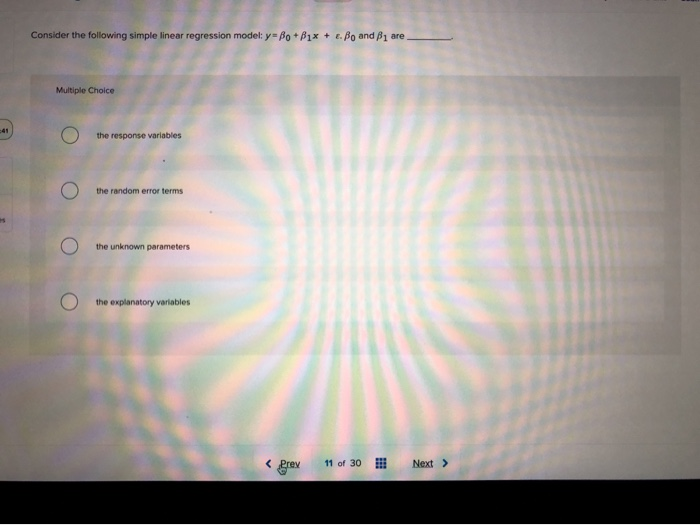Consider the following simple linear regression model: y=Po+P1x Po and B1 are Multiple Choice 41 the response variables the random error terms the unknown parameters the explanatory variables 11 of 30 Prev Next

• ### QUESTION 20 In what parts of a linear programming formulation do the decision variables appear? In...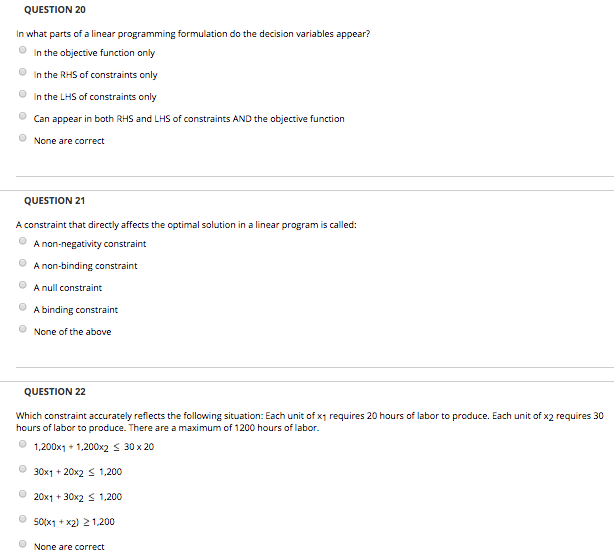QUESTION 20 In what parts of a linear programming formulation do the decision variables appear? In the objective function only In the RHS of constraints only In the LHS of constraints only Can appear in both RHS and LHS of constraints AND the objective function None are correct QUESTION 21 A constraint that directly affects the optimal solution in a linear program is called A non-binding constraint A null constraint A binding constraint None of the above QUESTION 22 Which...

• ### perfecting a rich decision-making background, modeling with actual simulation data, and solving (mainly linear programming models...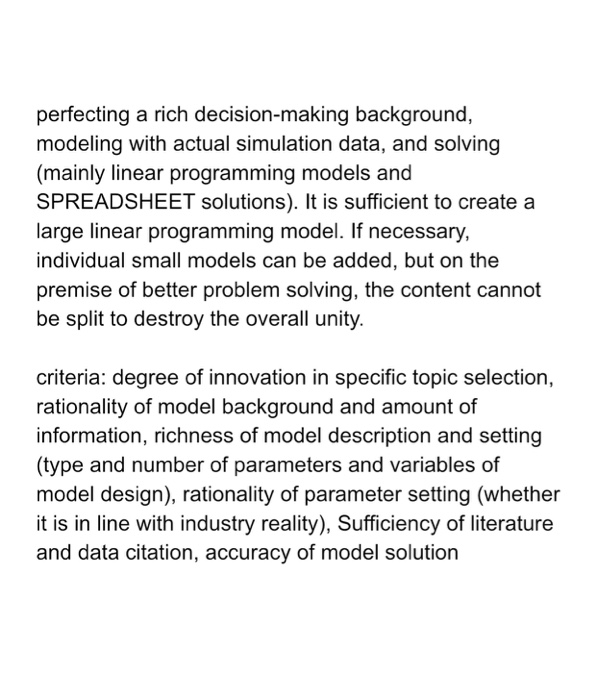perfecting a rich decision-making background, modeling with actual simulation data, and solving (mainly linear programming models and SPREADSHEET solutions). It is sufficient to create a large linear programming model. If necessary, individual small models can be added, but on the premise of better problem solving, the content cannot be split to destroy the overall unity. criteria: degree of innovation in specific topic selection, rationality of model background and amount of information, richness of model description and setting (type and number...

• ### Which of these is a measure summarizing the overall past performance of an investment?

Which of these is a measure summarizing the overall past performance of an investment?

• ### Linear Programming Corner Point Coordiantes and Optimal Solution

Given this linear programming model:Maximize Z = 10X1 + 16X2 (profit)Subject toa. 8X1 + 20X2 ≤ 120b. 25X1 + 20X2 ≤ 2001. Graph the constraints2. Shade in feasible solution space.3. Plot the objective function and determine the optimal point on the graph4. Use simultaneous equations to determine the optimal values of the decision variables and the maximum value of the objective function.5. What would be the optimal values be if the objective function had been 6X1 + 3X2Results4. X1 =...

• ### Can someone please answer? Thank you! Write a minimum of 250 words for each of the...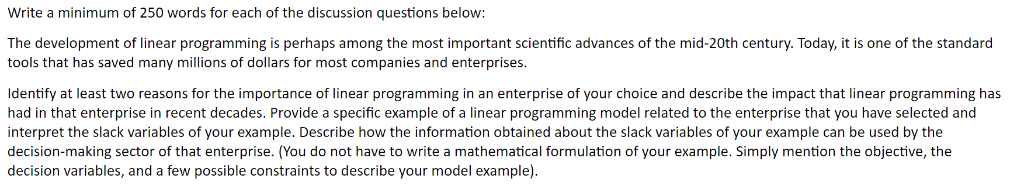Can someone please answer? Thank you! Write a minimum of 250 words for each of the discussion questions below: The development of linear programming is perhaps among the most important scientific advances of the mid-20th century. Today, it is one of the standard tools that has saved many millions of dollars for most companies and enterprises. Identify at least two reasons for the importance of linear programming in an enterprise of your choice and describe the impact that linear programming...

• ### Assignment 1. Linear Programming Case Study Your instructor will assign a linear programming project for this assignment...

Assignment 1. Linear Programming Case Study Your instructor will assign a linear programming project for this assignment according to the following specifications. It will be a problem with at least three (3) constraints and at least two (2) decision variables. The problem will be bounded and feasible. It will also have a single optimum solution (in other words, it won’t have alternate optimal solutions). The problem will also include a component that involves sensitivity analysis and the use of the...

• ### Consider the following linear programming model Max          2X1 + 3X2 Subject to:                   X1 + X2 &n

Consider the following linear programming model Max          2X1 + 3X2 Subject to:                   X1 + X2                   X1 ≥ 2                   X1, X2  ≥  0 This linear programming model has: A. Infeasible solution B. Unique solution C. Unbounded Solution D. Alternate optimal solution E. Redundant constraints